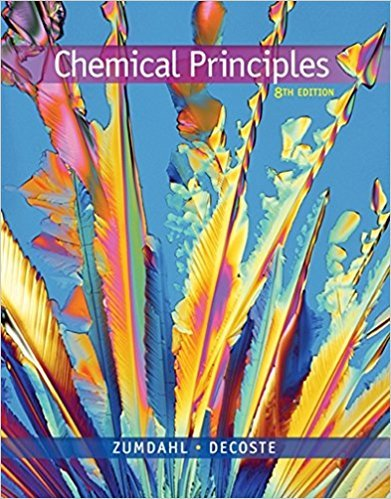×
Get Full Access to Chemical Principles - 8 Edition - Chapter 15 - Problem 15.127
Get Full Access to Chemical Principles - 8 Edition - Chapter 15 - Problem 15.127

×

# Consider the following reaction: CH3X 1 Y 88n CH3Y 1 X AtISBN: 9781305581982 176

## Solution for problem 15.127 Chapter 15

Chemical Principles | 8th Edition

• Textbook Solutions
• 2901 Step-by-step solutions solved by professors and subject experts
• Get 24/7 help from StudySoup virtual teaching assistantsChemical Principles | 8th Edition

4 5 1 276 Reviews
17
3
Problem 15.127

Consider the following reaction: CH3X 1 Y 88n CH3Y 1 X At 258C the following two experiments were run, yielding the following data: Experiment 1: [Y]0 5 3.0 M [CH3X] Time (h) 7.08 3 1023 M 1.0 4.52 3 1023 M 1.5 2.23 3 1023 M 2.3 4.76 3 1024 M 4.0 8.44 3 1025 M 5.7 2.75 3 1025 M 7.0 Experiment 2: [Y]0 5 4.5 M [CH3X] Time (h) 4.50 3 1023 M 0 1.70 3 1023 M 1.0 4.19 3 1024 M 2.5 1.11 3 1024 M 4.0 2.81 3 1025 M 5.5 Experiments were also run at 858C. The value of the rate constant at 858C was found to be 7.88 3 108 (with the time in units of hours), where [CH3X]0 5 1.0 3 1022 M and [Y]0 5 3.0 M. a. Determine the rate law and the value of k for this reaction at 258C. b. Determine the half-life at 858C. c. Determine Ea for the reaction. d. Given that the COX bond energy is known to be about 325 kJ/mol, suggest a mechanism that explains the results in parts a and c.

Step-by-Step Solution:
Step 1 of 3

C101 Elementary Chemistry IUPUI Week 2 Temperature and T emperature Scales 3 Scales:  Celsius ­ scale of temperature in which water freezes at 0° and boils at 100° under standard conditions.  Fahrenheit ­ scale of temperature on which water freezes at 32° and boils at 212° under standard conditions.  Kelvin ­ the SI base unit of thermodynamic temperature, equal in magnitude to the degree Celsius. Conversions: C= 5(F−32) 9 F= C+32 5 K=C+273 Celsius and Fahrenheit are bo

Step 2 of 3

Step 3 of 3

##### ISBN: 9781305581982

This full solution covers the following key subjects: . This expansive textbook survival guide covers 20 chapters, and 2754 solutions. Since the solution to 15.127 from 15 chapter was answered, more than 461 students have viewed the full step-by-step answer. Chemical Principles was written by and is associated to the ISBN: 9781305581982. This textbook survival guide was created for the textbook: Chemical Principles, edition: 8. The answer to “Consider the following reaction: CH3X 1 Y 88n CH3Y 1 X At 258C the following two experiments were run, yielding the following data: Experiment 1: [Y]0 5 3.0 M [CH3X] Time (h) 7.08 3 1023 M 1.0 4.52 3 1023 M 1.5 2.23 3 1023 M 2.3 4.76 3 1024 M 4.0 8.44 3 1025 M 5.7 2.75 3 1025 M 7.0 Experiment 2: [Y]0 5 4.5 M [CH3X] Time (h) 4.50 3 1023 M 0 1.70 3 1023 M 1.0 4.19 3 1024 M 2.5 1.11 3 1024 M 4.0 2.81 3 1025 M 5.5 Experiments were also run at 858C. The value of the rate constant at 858C was found to be 7.88 3 108 (with the time in units of hours), where [CH3X]0 5 1.0 3 1022 M and [Y]0 5 3.0 M. a. Determine the rate law and the value of k for this reaction at 258C. b. Determine the half-life at 858C. c. Determine Ea for the reaction. d. Given that the COX bond energy is known to be about 325 kJ/mol, suggest a mechanism that explains the results in parts a and c.” is broken down into a number of easy to follow steps, and 189 words. The full step-by-step solution to problem: 15.127 from chapter: 15 was answered by , our top Chemistry solution expert on 12/23/17, 04:53PM.

Unlock Textbook Solution